### Learning Objectives

In this section, you will:

• Recognize characteristics of parabolas.
• Understand how the graph of a parabola is related to its quadratic function.
• Determine a quadratic function’s minimum or maximum value.
• Solve problems involving a quadratic function’s minimum or maximum value.Figure 1. An array of satellite dishes. (credit: Matthew Colvin de Valle, Flickr)

Curved antennas, such as the ones shown in (Figure), are commonly used to focus microwaves and radio waves to transmit television and telephone signals, as well as satellite and spacecraft communication. The cross-section of the antenna is in the shape of a parabola, which can be described by a quadratic function.

In this section, we will investigate quadratic functions, which frequently model problems involving area and projectile motion. Working with quadratic functions can be less complex than working with higher degree functions, so they provide a good opportunity for a detailed study of function behavior.

### Recognizing Characteristics of Parabolas

The graph of a quadratic function is a U-shaped curve called a parabola. One important feature of the graph is that it has an extreme point, called the vertex. If the parabola opens up, the vertex represents the lowest point on the graph, or the minimum value of the quadratic function. If the parabola opens down, the vertex represents the highest point on the graph, or the maximum value. In either case, the vertex is a turning point on the graph. The graph is also symmetric with a vertical line drawn through the vertex, called the axis of symmetry. These features are illustrated in (Figure).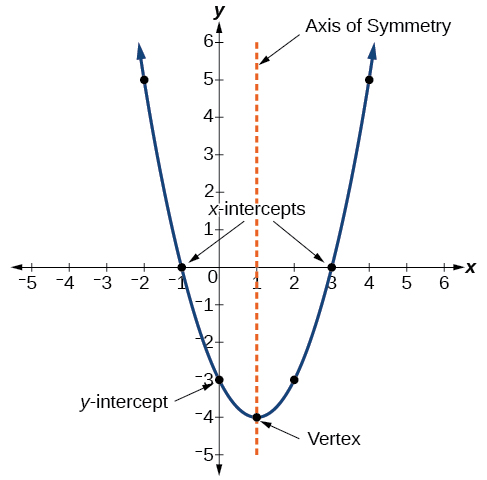Figure 2.

The y-intercept is the point at which the parabola crosses the y-axis. The x-intercepts are the points at which the parabola crosses the x-axis. If they exist, the x-intercepts represent the zeros, or roots, of the quadratic function, the values of$\,x\,$at which$\,y=0.$

### Identifying the Characteristics of a Parabola

Determine the vertex, axis of symmetry, zeros, and$\,y\text{-}$intercept of the parabola shown in (Figure).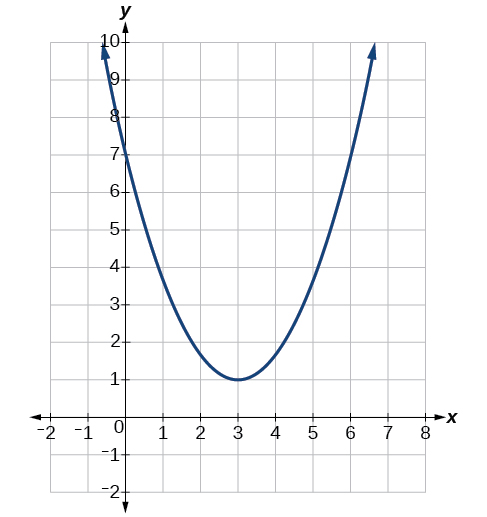Figure 3.

### Understanding How the Graphs of Parabolas are Related to Their Quadratic Functions

The general form of a quadratic function presents the function in the form

$f\left(x\right)=a{x}^{2}+bx+c$

where$\,a,b,\,$and$\,c\,$are real numbers and$\,a\ne 0.\,$If$\,a>0,\,$the parabola opens upward. If$\,a<0,\,$the parabola opens downward. We can use the general form of a parabola to find the equation for the axis of symmetry.

The axis of symmetry is defined by$\,x=-\frac{b}{2a}.\,$If we use the quadratic formula,$\,x=\frac{-b±\sqrt{{b}^{2}-4ac}}{2a},\,$to solve$\,a{x}^{2}+bx+c=0\,$for the$\,x\text{-}$intercepts, or zeros, we find the value of$\,x\,$halfway between them is always$\,x=-\frac{b}{2a},\,$the equation for the axis of symmetry.

(Figure) represents the graph of the quadratic function written in general form as$\,y={x}^{2}+4x+3.\,$In this form,$\,a=1,b=4,\,$and$\,c=3.\,$Because$\,a>0,\,$the parabola opens upward. The axis of symmetry is$\,x=-\frac{4}{2\left(1\right)}=-2.\,$This also makes sense because we can see from the graph that the vertical line$\,x=-2\,$divides the graph in half. The vertex always occurs along the axis of symmetry. For a parabola that opens upward, the vertex occurs at the lowest point on the graph, in this instance,$\,\left(-2,-1\right).\,$The$\,x\text{-}$intercepts, those points where the parabola crosses the$\,x\text{-}$axis, occur at$\,\left(-3,0\right)\,$and$\,\left(-1,0\right).$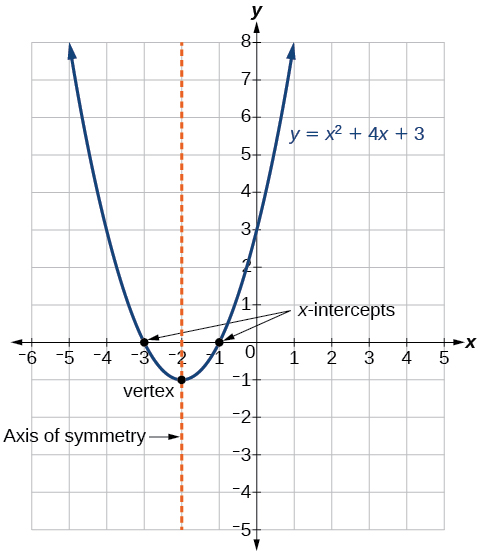Figure 4.

The standard form of a quadratic function presents the function in the form

$f\left(x\right)=a{\left(x-h\right)}^{2}+k$

where$\,\left(h,\text{ }k\right)\,$is the vertex. Because the vertex appears in the standard form of the quadratic function, this form is also known as the vertex form of a quadratic function.

As with the general form, if$\,a>0,\,$the parabola opens upward and the vertex is a minimum. If$\,a<0,\,$the parabola opens downward, and the vertex is a maximum. (Figure) represents the graph of the quadratic function written in standard form as$\,y=-3{\left(x+2\right)}^{2}+4.\,$Since$\,x–h=x+2\,$in this example,$\,h=–2.\,$In this form,$\,a=-3,h=-2,\,$and$\,k=4.\,$Because$\,a<0,\,$the parabola opens downward. The vertex is at$\,\left(-2,\text{ 4}\right).$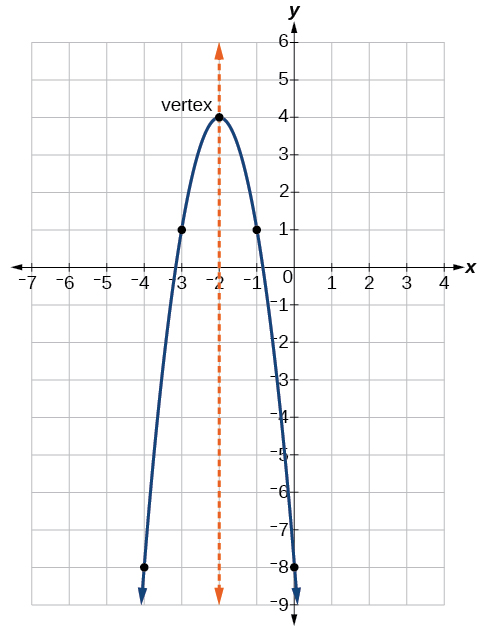Figure 5.

The standard form is useful for determining how the graph is transformed from the graph of$\,y={x}^{2}.\,$(Figure) is the graph of this basic function.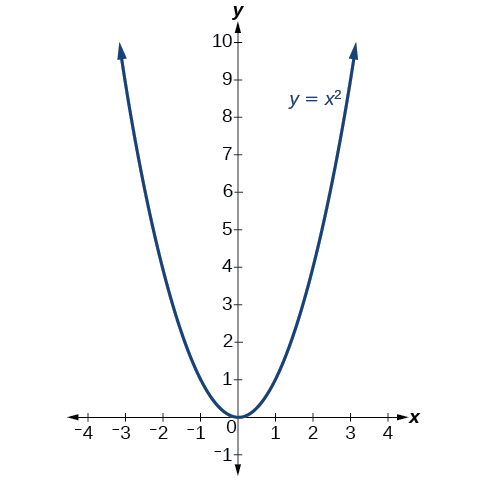Figure 6.

If$\,k>0,\,$the graph shifts upward, whereas if$\,k<0,\,$the graph shifts downward. In (Figure),$\,k>0,\,$so the graph is shifted 4 units upward. If$\,h>0,\,$the graph shifts toward the right and if$\,h<0,\,$the graph shifts to the left. In (Figure),$\,h<0,\,$so the graph is shifted 2 units to the left. The magnitude of$\,a\,$indicates the stretch of the graph. If$|a|>1,$ the point associated with a particular$\,x\text{-}$value shifts farther from the x-axis, so the graph appears to become narrower, and there is a vertical stretch. But if$\,|a|<1,\,$the point associated with a particular$\,x\text{-}$value shifts closer to the x-axis, so the graph appears to become wider, but in fact there is a vertical compression. In (Figure),$\,|a|>1,\,$so the graph becomes narrower.

The standard form and the general form are equivalent methods of describing the same function. We can see this by expanding out the general form and setting it equal to the standard form.

$\begin{array}{ccc}\hfill a{\left(x-h\right)}^{2}+k& =& a{x}^{2}+bx+c\hfill \\ \hfill a{x}^{2}-2ahx+\left(a{h}^{2}+k\right)& =& a{x}^{2}+bx+c\hfill \end{array}$

For the linear terms to be equal, the coefficients must be equal.

$–2ah=b,\text{ so }h=-\frac{b}{2a}$

This is the axis of symmetry we defined earlier. Setting the constant terms equal:

$\begin{array}{ccc}\hfill a{h}^{2}+k& =& c\hfill \\ \hfill k& =& c-a{h}^{2}\hfill \\ & =& c-a-{\left(\frac{b}{2a}\right)}^{2}\hfill \\ & =& c-\frac{{b}^{2}}{4a}\hfill \end{array}$

In practice, though, it is usually easier to remember that k is the output value of the function when the input is$\,h,\,$so$\,f\left(h\right)=k.$

A quadratic function is a polynomial function of degree two. The graph of a quadratic function is a parabola.

The general form of a quadratic function is$\,f\left(x\right)=a{x}^{2}+bx+c\,$where$\,a,b,\,$and$\,c\,$are real numbers and$\,a\ne 0.$

The standard form of a quadratic function is$\,f\left(x\right)=a{\left(x-h\right)}^{2}+k\,$where$\,a\ne 0.$

The vertex$\,\left(h,k\right)\,$is located at

$h=–\frac{b}{2a},\text{ }k=f\left(h\right)=f\left(\frac{-b}{2a}\right)$

### How To

Given a graph of a quadratic function, write the equation of the function in general form.

1. Identify the horizontal shift of the parabola; this value is$\,h.\,$Identify the vertical shift of the parabola; this value is$\,k.$
2. Substitute the values of the horizontal and vertical shift for$\,h\,$and$\,k.\,$in the function$\,f\left(x\right)=a{\left(x–h\right)}^{2}+k.$
3. Substitute the values of any point, other than the vertex, on the graph of the parabola for$\,x\,$and$\,f\left(x\right).$
4. Solve for the stretch factor,$\,|a|.$
5. Expand and simplify to write in general form.

### Writing the Equation of a Quadratic Function from the Graph

Write an equation for the quadratic function$\,g\,$in (Figure) as a transformation of$\,f\left(x\right)={x}^{2},\,$and then expand the formula, and simplify terms to write the equation in general form.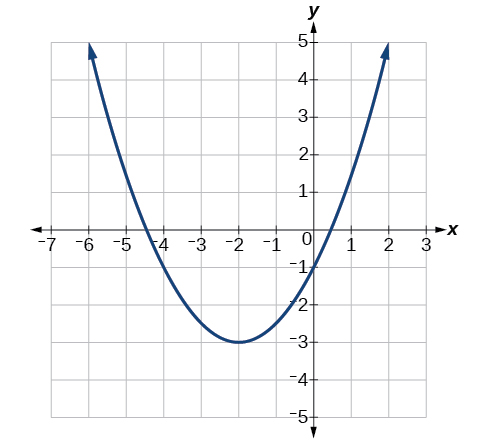Figure 7.

#### Analysis

We can check our work using the table feature on a graphing utility. First enter$\,\text{Y1}=\frac{1}{2}{\left(x+2\right)}^{2}-3.\,$Next, select$\,\text{TBLSET,}\,$then use$\,\text{TblStart}=–6\,$and$\,\Delta \text{Tbl = 2,}\,$and select$\,\text{TABLE}\text{.}\,$See (Figure).

 $x$ –6 –4 –2 0 2 $y$ 5 –1 –3 –1 5

The ordered pairs in the table correspond to points on the graph.

### Try It

A coordinate grid has been superimposed over the quadratic path of a basketball in (Figure). Find an equation for the path of the ball. Does the shooter make the basket?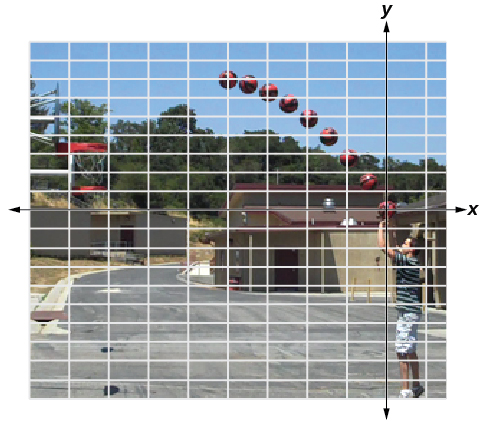Figure 8. (credit: modification of work by Dan Meyer)

### How To

Given a quadratic function in general form, find the vertex of the parabola.

1. Identify$\,a, b, \text{and} c.$
2. Find$\,h,\,$the x-coordinate of the vertex, by substituting$\,a\,$and$\,b\,$into$\,h=–\frac{b}{2a}.$
3. Find$\,k,\,$the y-coordinate of the vertex, by evaluating$\,k=f\left(h\right)=f\left(-\frac{b}{2a}\right).$

### Finding the Vertex of a Quadratic Function

Find the vertex of the quadratic function$\,f\left(x\right)=2{x}^{2}–6x+7.\,$Rewrite the quadratic in standard form (vertex form).

#### Analysis

One reason we may want to identify the vertex of the parabola is that this point will inform us where the maximum or minimum value of the output occurs,$\,k,$and where it occurs,$\,x.$

### Try It

Given the equation$\,g\left(x\right)=13+{x}^{2}-6x,$ write the equation in general form and then in standard form.

### Finding the Domain and Range of a Quadratic Function

Any number can be the input value of a quadratic function. Therefore, the domain of any quadratic function is all real numbers. Because parabolas have a maximum or a minimum point, the range is restricted. Since the vertex of a parabola will be either a maximum or a minimum, the range will consist of all y-values greater than or equal to the y-coordinate at the turning point or less than or equal to the y-coordinate at the turning point, depending on whether the parabola opens up or down.

### Domain and Range of a Quadratic Function

The domain of any quadratic function is all real numbers unless the context of the function presents some restrictions.

The range of a quadratic function written in general form$\,f\left(x\right)=a{x}^{2}+bx+c\,$with a positive$\,a\,$value is$\,f\left(x\right)\ge f\left(-\frac{b}{2a}\right),\,$or$\,\left[f\left(-\frac{b}{2a}\right),\infty \right);\,$the range of a quadratic function written in general form with a negative$\,a\,$value is$\,f\left(x\right)\le f\left(-\frac{b}{2a}\right),\,$or$\,\left(-\infty ,f\left(-\frac{b}{2a}\right)\right].$

The range of a quadratic function written in standard form$\,f\left(x\right)=a{\left(x-h\right)}^{2}+k\,$with a positive$\,a\,$value is$\,f\left(x\right)\ge k;\,$the range of a quadratic function written in standard form with a negative$\,a\,$value is$\,f\left(x\right)\le k.$

### How To

Given a quadratic function, find the domain and range.

1. Identify the domain of any quadratic function as all real numbers.
2. Determine whether$\,a\,$is positive or negative. If$\,a\,$is positive, the parabola has a minimum. If$\,a\,$is negative, the parabola has a maximum.
3. Determine the maximum or minimum value of the parabola,$\,k.$
4. If the parabola has a minimum, the range is given by$\,f\left(x\right)\ge k,\,$or$\,\left[k,\infty \right).\,$If the parabola has a maximum, the range is given by$\,f\left(x\right)\le k,\,$or$\,\left(-\infty ,k\right].$

### Finding the Domain and Range of a Quadratic Function

Find the domain and range of$\,f\left(x\right)=-5{x}^{2}+9x-1.$

### Try It

Find the domain and range of$\,f\left(x\right)=2{\left(x-\frac{4}{7}\right)}^{2}+\frac{8}{11}.$

### Determining the Maximum and Minimum Values of Quadratic Functions

The output of the quadratic function at the vertex is the maximum or minimum value of the function, depending on the orientation of the parabola. We can see the maximum and minimum values in (Figure).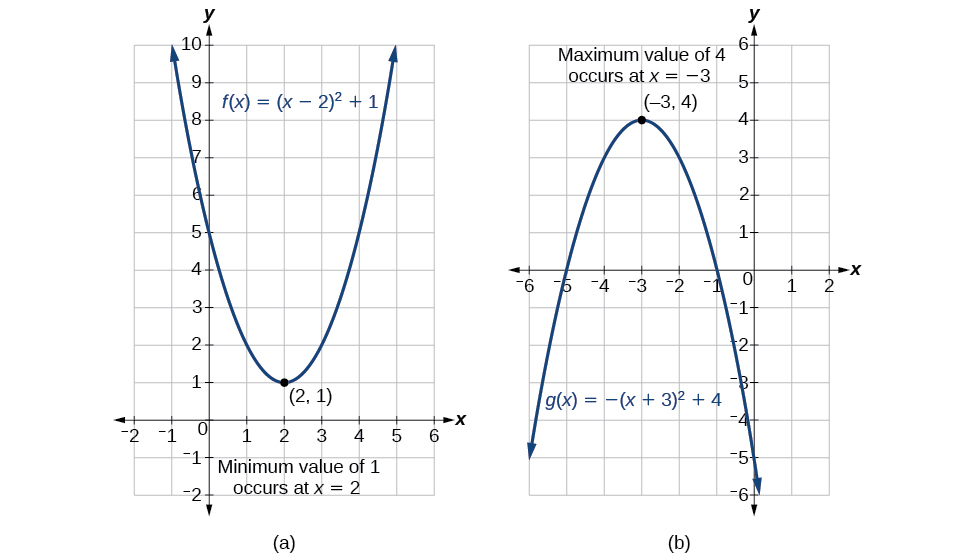Figure 9.

There are many real-world scenarios that involve finding the maximum or minimum value of a quadratic function, such as applications involving area and revenue.

### Finding the Maximum Value of a Quadratic Function

A backyard farmer wants to enclose a rectangular space for a new garden within her fenced backyard. She has purchased 80 feet of wire fencing to enclose three sides, and she will use a section of the backyard fence as the fourth side.

1. Find a formula for the area enclosed by the fence if the sides of fencing perpendicular to the existing fence have length$\,L.$
2. What dimensions should she make her garden to maximize the enclosed area?

#### Analysis

This problem also could be solved by graphing the quadratic function. We can see where the maximum area occurs on a graph of the quadratic function in (Figure).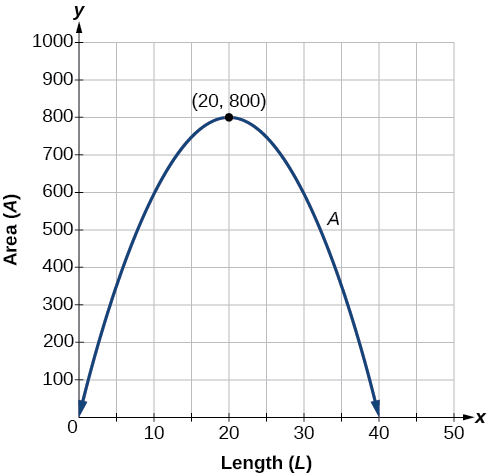Figure 11.

### How To

Given an application involving revenue, use a quadratic equation to find the maximum.

1. Write a quadratic equation for a revenue function.
2. Find the vertex of the quadratic equation.
3. Determine the y-value of the vertex.

### Finding Maximum Revenue

The unit price of an item affects its supply and demand. That is, if the unit price goes up, the demand for the item will usually decrease. For example, a local newspaper currently has 84,000 subscribers at a quarterly charge of $30. Market research has suggested that if the owners raise the price to$32, they would lose 5,000 subscribers. Assuming that subscriptions are linearly related to the price, what price should the newspaper charge for a quarterly subscription to maximize their revenue?

#### Analysis

This could also be solved by graphing the quadratic as in (Figure). We can see the maximum revenue on a graph of the quadratic function.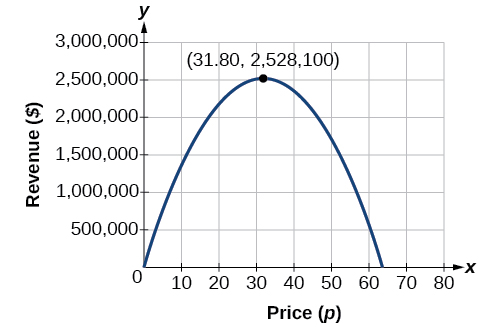Figure 12.

#### Finding the x– and y-Intercepts of a Quadratic Function

Much as we did in the application problems above, we also need to find intercepts of quadratic equations for graphing parabolas. Recall that we find the$\,y\text{-}$intercept of a quadratic by evaluating the function at an input of zero, and we find the$\,x\text{-}$intercepts at locations where the output is zero. Notice in (Figure) that the number of$\,x\text{-}$intercepts can vary depending upon the location of the graph.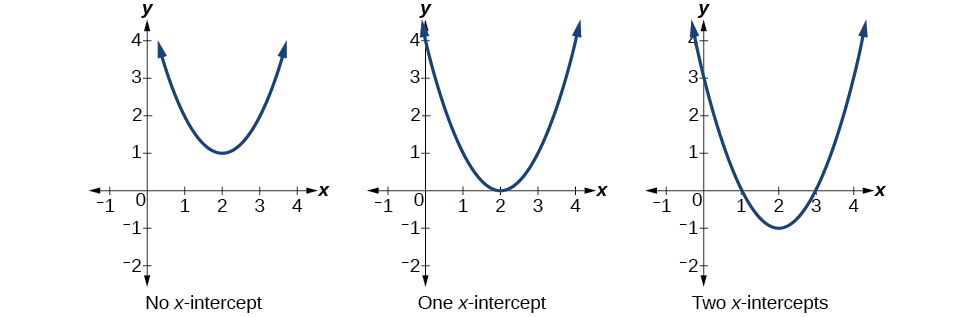Figure 13. Number of x-intercepts of a parabola

### How To

Given a quadratic function$\,f\left(x\right),\,$find the$\,y\text{-}$ and x-intercepts.

1. Evaluate$\,f\left(0\right)\,$to find the y-intercept.
2. Solve the quadratic equation$\,f\left(x\right)=0\,$to find the x-intercepts.

### Finding the y– and x-Intercepts of a Parabola

Find the y– and x-intercepts of the quadratic$\,f\left(x\right)=3{x}^{2}+5x-2.$

#### Analysis

By graphing the function, we can confirm that the graph crosses the y-axis at$\,\left(0,-2\right).\,$We can also confirm that the graph crosses the x-axis at$\,\left(\frac{1}{3},0\right)\,$and$\,\left(-2,0\right).$See (Figure)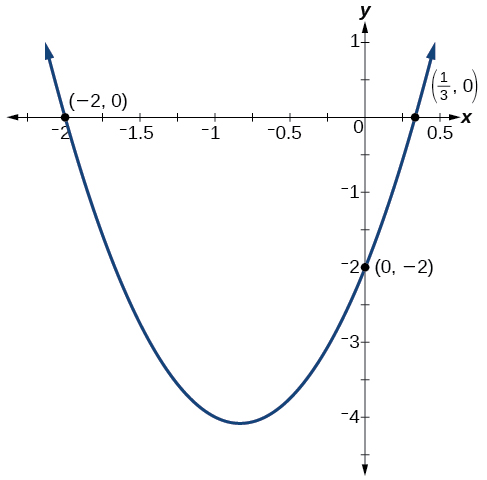Figure 14.

#### Rewriting Quadratics in Standard Form

In (Figure), the quadratic was easily solved by factoring. However, there are many quadratics that cannot be factored. We can solve these quadratics by first rewriting them in standard form.

### How To

Given a quadratic function, find the$\,x\text{-}$intercepts by rewriting in standard form.

1. Substitute$\,a\,$and$\,b\,$into$\,h=-\frac{b}{2a}.$
2. Substitute$\,x=h\,$into the general form of the quadratic function to find$\,k.$
3. Rewrite the quadratic in standard form using$\,h\,$and$\,k.$
4. Solve for when the output of the function will be zero to find the$\,x\text{-}$intercepts.

### Finding the x-Intercepts of a Parabola

Find the$\,x\text{-}$intercepts of the quadratic function$\,f\left(x\right)=2{x}^{2}+4x-4.$

#### Analysis

We could have achieved the same results using the quadratic formula. Identify$\,a=2,b=4\,$and$\,c=-4.$

$\begin{array}{ccc}\hfill x& =& \frac{-b±\sqrt{{b}^{2}-4ac}}{2a}\hfill \\ & =& \frac{-4±\sqrt{{4}^{2}-4\left(2\right)\left(-4\right)}}{2\left(2\right)}\hfill \\ & =& \frac{-4±\sqrt{48}}{4}\hfill \\ & =& \frac{-4±\sqrt{3\left(16\right)}}{4}\hfill \\ & =& -1±\sqrt{3}\hfill \end{array}$

So the x-intercepts occur at$\,\left(-1-\sqrt{3},0\right)\,$and$\,\left(-1+\sqrt{3},0\right).$

### Try It

In a Try It, we found the standard and general form for the function$\,g\left(x\right)=13+{x}^{2}-6x.\,$Now find the y– and x-intercepts (if any).

### Applying the Vertex and x-Intercepts of a Parabola

A ball is thrown upward from the top of a 40 foot high building at a speed of 80 feet per second. The ball’s height above ground can be modeled by the equation$\,H\left(t\right)=-16{t}^{2}+80t+40.$

1. When does the ball reach the maximum height?
2. What is the maximum height of the ball?
3. When does the ball hit the ground?

### Try It

A rock is thrown upward from the top of a 112-foot high cliff overlooking the ocean at a speed of 96 feet per second. The rock’s height above ocean can be modeled by the equation$\,H\left(t\right)=-16{t}^{2}+96t+112.$

1. When does the rock reach the maximum height?
2. What is the maximum height of the rock?
3. When does the rock hit the ocean?

Access these online resources for additional instruction and practice with quadratic equations.

### Key Equations

 general form of a quadratic function $f\left(x\right)=a{x}^{2}+bx+c$ standard form of a quadratic function $f\left(x\right)=a{\left(x-h\right)}^{2}+k$

### Key Concepts

• A polynomial function of degree two is called a quadratic function.
• The graph of a quadratic function is a parabola. A parabola is a U-shaped curve that can open either up or down.
• The axis of symmetry is the vertical line passing through the vertex. The zeros, or$\,x\text{-}$intercepts, are the points at which the parabola crosses the$\,x\text{-}$axis. The$\,y\text{-}$intercept is the point at which the parabola crosses the$\,y\text{-}$axis. See (Figure), (Figure), and (Figure).
• Quadratic functions are often written in general form. Standard or vertex form is useful to easily identify the vertex of a parabola. Either form can be written from a graph. See (Figure).
• The vertex can be found from an equation representing a quadratic function. See (Figure).
• The domain of a quadratic function is all real numbers. The range varies with the function. See (Figure).
• A quadratic function’s minimum or maximum value is given by the$\,y\text{-}$value of the vertex.
• The minimum or maximum value of a quadratic function can be used to determine the range of the function and to solve many kinds of real-world problems, including problems involving area and revenue. See (Figure) and (Figure).
• The vertex and the intercepts can be identified and interpreted to solve real-world problems. See (Figure).

### Section Exercises

#### Verbal

How can the vertex of a parabola be used in solving real-world problems?

Explain why the condition of$\,a\ne 0\,$is imposed in the definition of the quadratic function.

What is another name for the standard form of a quadratic function?

What two algebraic methods can be used to find the horizontal intercepts of a quadratic function?

#### Algebraic

For the following exercises, rewrite the quadratic functions in standard form and give the vertex.

$f\left(x\right)={x}^{2}-12x+32$

$g\left(x\right)={x}^{2}+2x-3$

$f\left(x\right)={x}^{2}-x$

$f\left(x\right)={x}^{2}+5x-2$

$h\left(x\right)=2{x}^{2}+8x-10$

$k\left(x\right)=3{x}^{2}-6x-9$

$f\left(x\right)=2{x}^{2}-6x$

$f\left(x\right)=3{x}^{2}-5x-1$

For the following exercises, determine whether there is a minimum or maximum value to each quadratic function. Find the value and the axis of symmetry.

$y\left(x\right)=2{x}^{2}+10x+12$

$f\left(x\right)=2{x}^{2}-10x+4$

$f\left(x\right)=-{x}^{2}+4x+3$

$f\left(x\right)=4{x}^{2}+x-1$

$h\left(t\right)=-4{t}^{2}+6t-1$

$f\left(x\right)=\frac{1}{2}{x}^{2}+3x+1$

$f\left(x\right)=-\frac{1}{3}{x}^{2}-2x+3$

For the following exercises, determine the domain and range of the quadratic function.

$f\left(x\right)={\left(x-3\right)}^{2}+2$

$f\left(x\right)=-2{\left(x+3\right)}^{2}-6$

$f\left(x\right)={x}^{2}+6x+4$

$f\left(x\right)=2{x}^{2}-4x+2$

$k\left(x\right)=3{x}^{2}-6x-9$

For the following exercises, use the vertex$\,\left(h,k\right)\,$and a point on the graph$\,\left(x,y\right)\,$to find the general form of the equation of the quadratic function.

$\left(h,k\right)=\left(2,0\right),\left(x,y\right)=\left(4,4\right)$

$\left(h,k\right)=\left(-2,-1\right),\left(x,y\right)=\left(-4,3\right)$

$\left(h,k\right)=\left(0,1\right),\left(x,y\right)=\left(2,5\right)$

$\left(h,k\right)=\left(2,3\right),\left(x,y\right)=\left(5,12\right)$

$\left(h,k\right)=\left(-5,3\right),\left(x,y\right)=\left(2,9\right)$

$\left(h,k\right)=\left(3,2\right),\left(x,y\right)=\left(10,1\right)$

$\left(h,k\right)=\left(0,1\right),\left(x,y\right)=\left(1,0\right)$

$\left(h,k\right)=\left(1,0\right),\left(x,y\right)=\left(0,1\right)$

#### Graphical

For the following exercises, sketch a graph of the quadratic function and give the vertex, axis of symmetry, and intercepts.

$f\left(x\right)={x}^{2}-2x$

$f\left(x\right)={x}^{2}-6x-1$

$f\left(x\right)={x}^{2}-5x-6$

$f\left(x\right)={x}^{2}-7x+3$

$f\left(x\right)=-2{x}^{2}+5x-8$

$f\left(x\right)=4{x}^{2}-12x-3$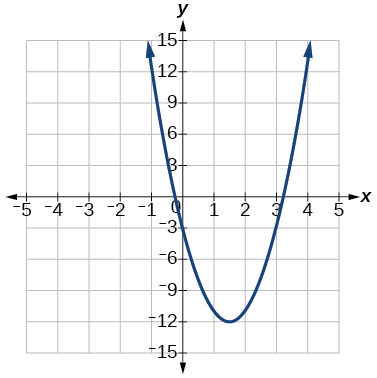For the following exercises, write the equation for the graphed quadratic function.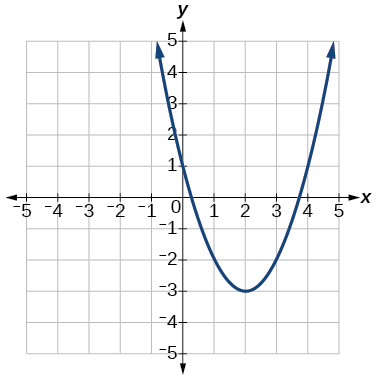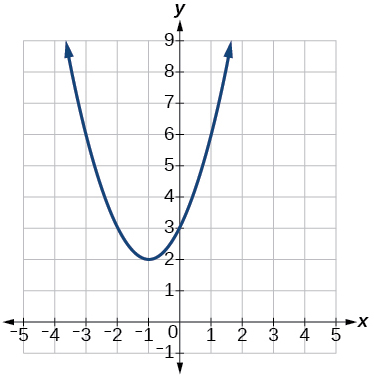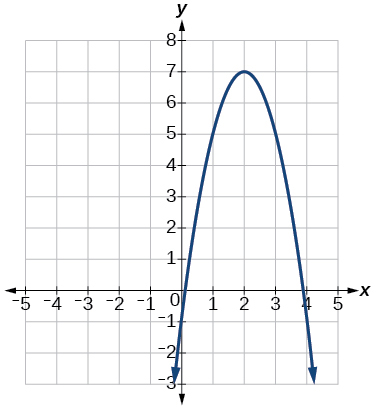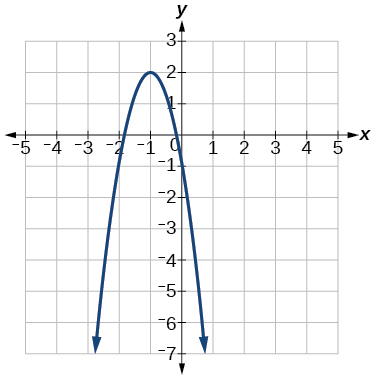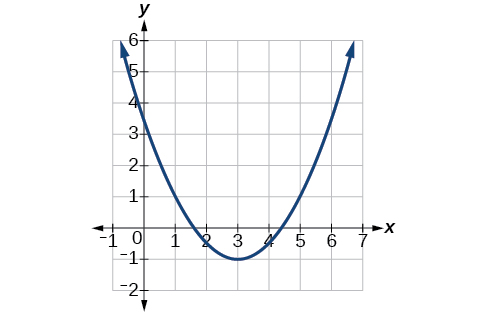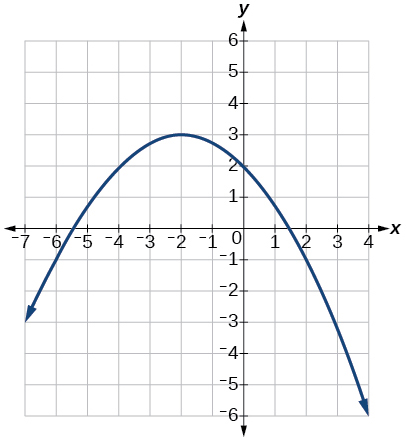#### Numeric

For the following exercises, use the table of values that represent points on the graph of a quadratic function. By determining the vertex and axis of symmetry, find the general form of the equation of the quadratic function.

 $x$ –2 –1 0 1 2 $y$ 5 2 1 2 5

 $x$ –2 –1 0 1 2 $y$ 1 0 1 4 9
 $x$ –2 –1 0 1 2 $y$ –2 1 2 1 –2

 $x$ –2 –1 0 1 2 $y$ –8 –3 0 1 0
 $x$ –2 –1 0 1 2 $y$ 8 2 0 2 8

#### Technology

For the following exercises, use a calculator to find the answer.

Graph on the same set of axes the functions$\,f\left(x\right)={x}^{2},f\left(x\right)=2{x}^{2},\text{ and }f\left(x\right)=\frac{1}{3}{x}^{2}.$

What appears to be the effect of changing the coefficient?

Graph on the same set of axes$\,f\left(x\right)={x}^{2},f\left(x\right)={x}^{2}+2\,$ and$\,f\left(x\right)={x}^{2},f\left(x\right)={x}^{2}+5\,$and$\,f\left(x\right)={x}^{2}-3.\,$ What appears to be the effect of adding a constant?

Graph on the same set of axes$\,f\left(x\right)={x}^{2},f\left(x\right)={\left(x-2\right)}^{2},f{\left(x-3\right)}^{2},\text{ and }f\left(x\right)={\left(x+4\right)}^{2}.$

What appears to be the effect of adding or subtracting those numbers?

The path of an object projected at a 45 degree angle with initial velocity of 80 feet per second is given by the function$\,h\left(x\right)=\frac{-32}{{\left(80\right)}^{2}}{x}^{2}+x\,$where$\,x\,$is the horizontal distance traveled and$\,h\left(x\right)\,$is the height in feet. Use the TRACE feature of your calculator to determine the height of the object when it has traveled 100 feet away horizontally.

A suspension bridge can be modeled by the quadratic function$\,h\left(x\right)=.0001{x}^{2}\,$with$\,-2000\le x\le 2000\,$where$\,|x|\,$is the number of feet from the center and$\,h\left(x\right)\,$is height in feet. Use the TRACE feature of your calculator to estimate how far from the center does the bridge have a height of 100 feet.

#### Extensions

For the following exercises, use the vertex of the graph of the quadratic function and the direction the graph opens to find the domain and range of the function.

Vertex$\,\left(1,-2\right),\,$opens up.

Vertex$\,\left(-1,2\right)\,$opens down.

Vertex$\,\left(-5,11\right),\,$opens down.

Vertex$\,\left(-100,100\right),\,$opens up.

For the following exercises, write the equation of the quadratic function that contains the given point and has the same shape as the given function.

Contains$\,\left(1,1\right)\,$and has shape of$\,f\left(x\right)=2{x}^{2}.\,$Vertex is on the$\,y\text{-}$axis.

Contains$\,\left(-1,4\right)\,$and has the shape of$\,f\left(x\right)=2{x}^{2}.\,$Vertex is on the$\,y\text{-}$axis.

Contains$\,\left(2,3\right)\,$and has the shape of$\,f\left(x\right)=3{x}^{2}.\,$Vertex is on the$\,y\text{-}$axis.

Contains$\,\left(1,-3\right)\,$and has the shape of$\,f\left(x\right)=-{x}^{2}.\,$Vertex is on the$\,y\text{-}$axis.

Contains$\,\left(4,3\right)\,$and has the shape of$\,f\left(x\right)=5{x}^{2}.\,$Vertex is on the$\,y\text{-}$axis.

Contains$\,\left(1,-6\right)\,$has the shape of$\,f\left(x\right)=3{x}^{2}.\,$Vertex has x-coordinate of$\,-1.$

#### Real-World Applications

Find the dimensions of the rectangular corral producing the greatest enclosed area given 200 feet of fencing.

Find the dimensions of the rectangular corral split into 2 pens of the same size producing the greatest possible enclosed area given 300 feet of fencing.

Find the dimensions of the rectangular corral producing the greatest enclosed area split into 3 pens of the same size given 500 feet of fencing.

Among all of the pairs of numbers whose sum is 6, find the pair with the largest product. What is the product?

Among all of the pairs of numbers whose difference is 12, find the pair with the smallest product. What is the product?

Suppose that the price per unit in dollars of a cell phone production is modeled by$\,p=\text{\}45-0.0125x,\,$where$\,x\,$is in thousands of phones produced, and the revenue represented by thousands of dollars is$\,R=x\cdot p.\,$Find the production level that will maximize revenue.

A rocket is launched in the air. Its height, in meters above sea level, as a function of time, in seconds, is given by$\,h\left(t\right)=-4.9{t}^{2}+229t+234.\,$Find the maximum height the rocket attains.

A ball is thrown in the air from the top of a building. Its height, in meters above ground, as a function of time, in seconds, is given by$\,h\left(t\right)=-4.9{t}^{2}+24t+8.\,$How long does it take to reach maximum height?

A soccer stadium holds 62,000 spectators. With a ticket price of $11, the average attendance has been 26,000. When the price dropped to$9, the average attendance rose to 31,000. Assuming that attendance is linearly related to ticket price, what ticket price would maximize revenue?

A farmer finds that if she plants 75 trees per acre, each tree will yield 20 bushels of fruit. She estimates that for each additional tree planted per acre, the yield of each tree will decrease by 3 bushels. How many trees should she plant per acre to maximize her harvest?

### Glossary

axis of symmetry
a vertical line drawn through the vertex of a parabola, that opens up or down, around which the parabola is symmetric; it is defined by$\,x=-\frac{b}{2a}.$
general form of a quadratic function
the function that describes a parabola, written in the form$\,f\left(x\right)=a{x}^{2}+bx+c$, where$\,a,b,\,$and$\,c\,$are real numbers and$\,a\ne 0.$
roots
in a given function, the values of$\,x\,$at which$\,y=0$, also called zeros
standard form of a quadratic function
the function that describes a parabola, written in the form$\,f\left(x\right)=a{\left(x-h\right)}^{2}+k$, where$\,\left(h,\text{ }k\right)\,$is the vertex
vertex
the point at which a parabola changes direction, corresponding to the minimum or maximum value of the quadratic function
vertex form of a quadratic function
another name for the standard form of a quadratic function
zeros
in a given function, the values of$\,x\,$at which$\,y=0$, also called roots